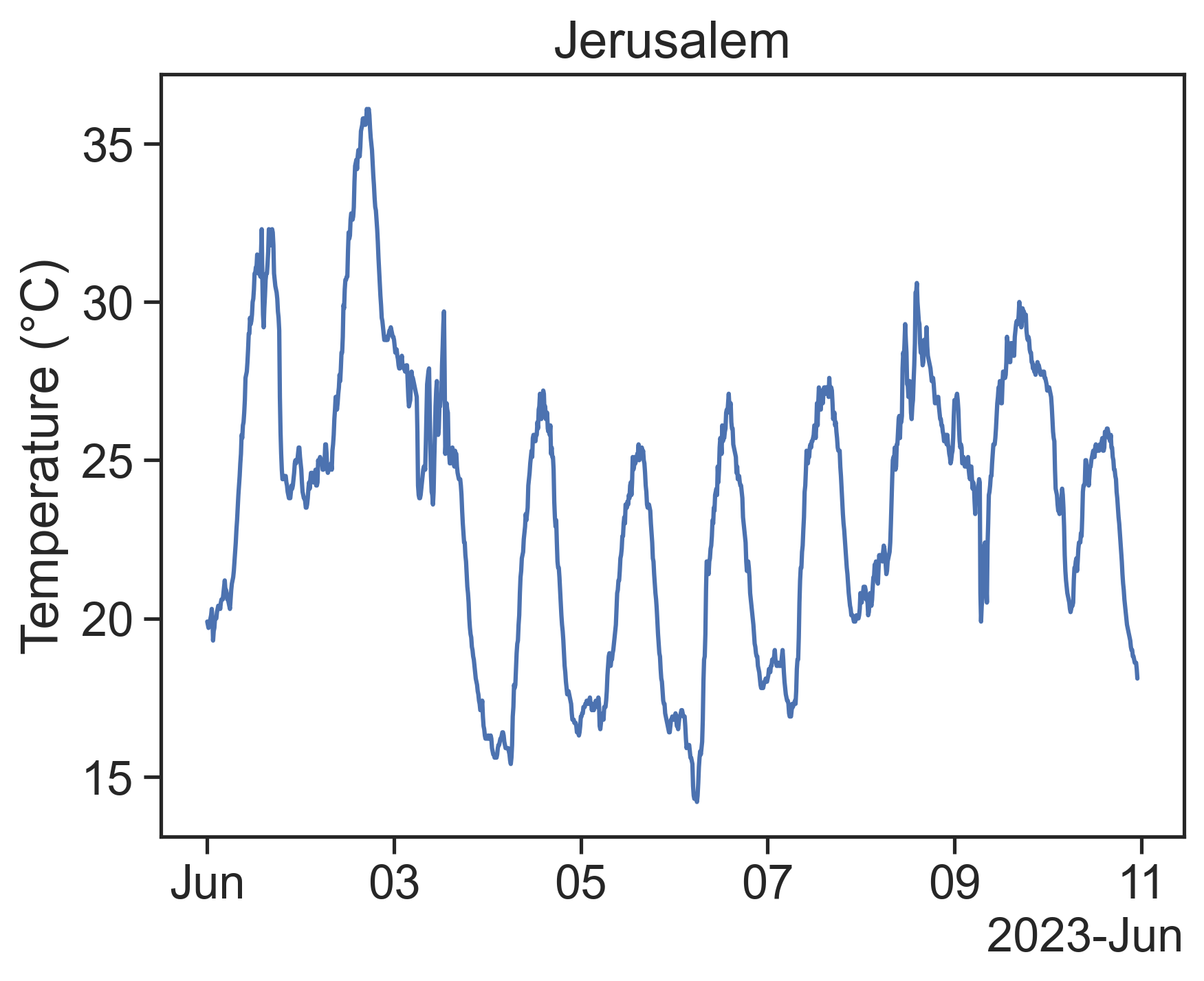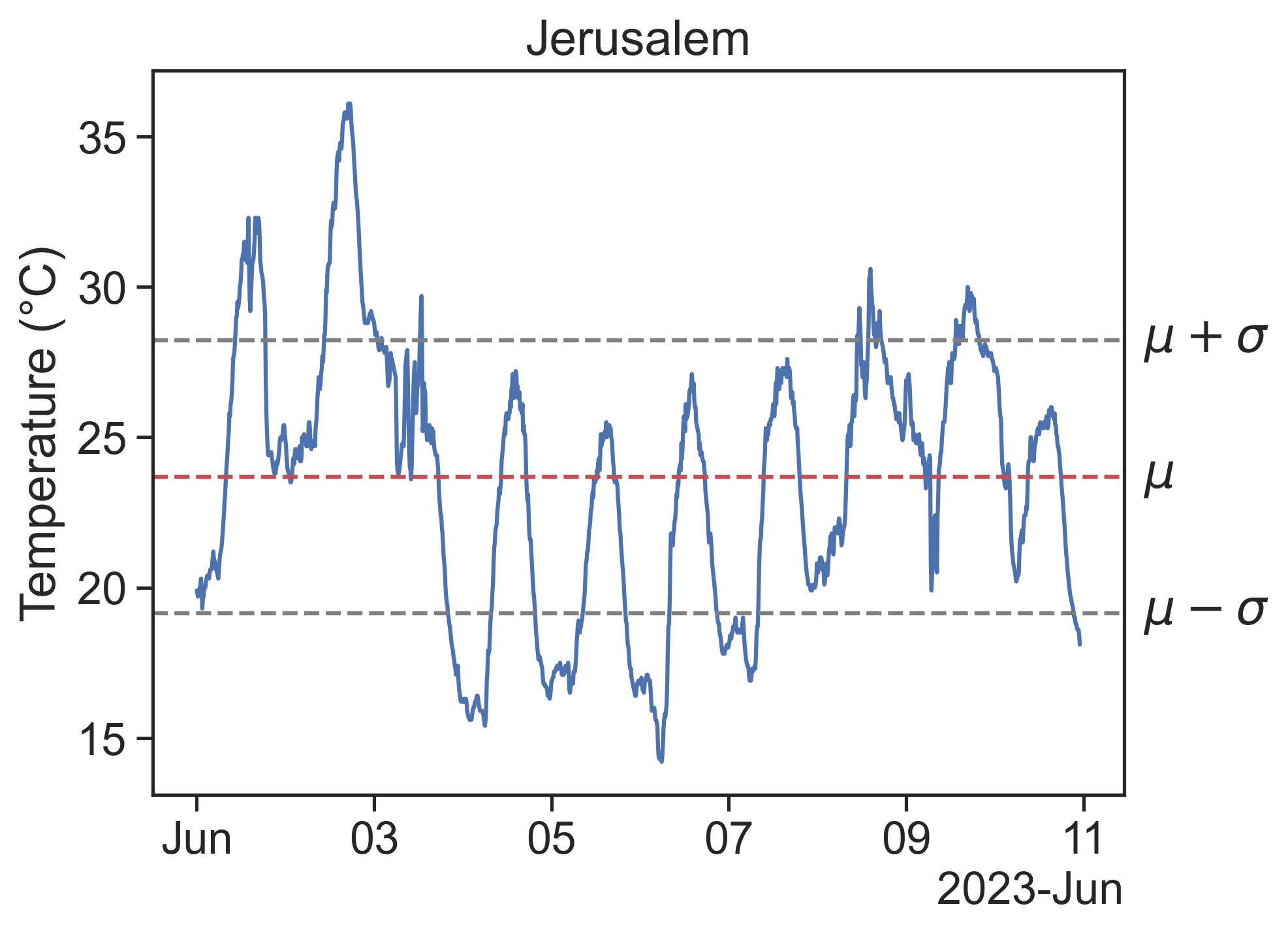# 20autocorrelation

See the temperatures for Jerusalem in a 4-day interval:## 20.1 question

If I know the temperature right now, what does that tell me about the temperature 10 minutes from now? How about 100 minutes? 1000 minutes?

To answer this, we need to talk about autocorrelation. Let’s start by introducing the necessary concepts.

## 20.2 mean and standard deviation

Let’s call our time series from above $$X$$, and its length $$N$$. Then:

\begin{aligned} \text{mean}& &\mu &= \frac{\displaystyle\sum_{i=1}^N X_i}{N} \\ \text{standard deviation}& &\sigma &= \sqrt{\frac{\displaystyle\sum_{i=1}^N (X_i-\mu)^2}{N}} \end{aligned}

The mean and standard deviation can be visualized thus:One last basic concept we need is the expected value: $E[X] = \sum_{i=1}^N X_i p_i$

For our time series, the probability $$p_i$$ that a given point $$X_i$$ is in the dataset is simply $$1/N$$, therefore the expectation becomes

$E[X] = \frac{\displaystyle\sum_{i=1}^N X_i}{N}$

## 20.3 autocorrelation

The autocorrelation of a time series $$X$$ is the answer to the following question:

if we shift $$X$$ by $$\tau$$ units, how similar will this be to the original signal?

In other words:

how correlated are $$X(t)$$ and $$X(t+\tau)$$?

Using the Pearson correlation coefficient

Pearson correlation coefficient between $$X$$ and $$Y$$: $\rho_{X,Y} = \frac{E\left[ (X - \mu_X)(X_Y - \mu_Y) \right]}{\sigma_X\sigma_Y}$

we get

$\rho_{XX}(\tau) = \frac{E\left[ (X_t - \mu)(X_{t+\tau} - \mu) \right]}{\sigma^2}$

A video is worth a billion words, so let’s see the autocorrelation in action:

• The autocorrelation for $$\tau=0$$ (zero shift) is always 1.